# Online Civil Engineering Test - Water Resources Engineering TestLoading Test...

Instruction:

• This is a FREE online test. DO NOT pay money to anyone to attend this test.
• Total number of questions : 20.
• Time alloted : 30 minutes.
• Each question carry 1 mark, no negative marks.
• DO NOT refresh the page.
• All the best :-).

1.

Pick up the correct statement from the following :

A.
 The portion of pellicular water which remains unutilised, is called hygroscopic waterB.
 The moisture content at which permanent wilting of plants takes place, is called the wilting pointC.
 The path of the water required to bring the soil moisture content of a soil up to its field capacity is called soil moisture deficiencyD.
 The moisture deficiency will be different at different pointsE.
 All the above.2.

According to Robert E. Horton, the equation of infiltration capacity curve, is (where letters carry their usual meanings)

A.
 f = fc (fo - fc) ektB.
 f = ft - (fo - fc) e-ktC.
 f = ft + (fo - fc) e-ktD.
 f = f + (fo - fc) ekt3.

Precipitation caused by lifting of an air mass due to the pressure difference, is called

A.
 cyclonic precipitationB.
 convective precipitationC.
 orographic precipitationD.
 none of these.4.

A recording type rain gauge

A.
 produces a mass curve of rain fallB.
 records the cumulative rainC.
 is sometimes called integrating rain gauge or continuous rain gaugeD.
 all the above.5.

For determination of average annual precipitation in a catchment basin, the best method is

A.
 Arithmetical methodB.
 Thiessen's mean methodC.
 Isohyetal methodD.
 None of these.6.

While calculating the average depth of annual precipitation in a catchment basin, importance to individual raingauge station is given in

A.
 Arithmetical methodB.
 Thiessen's mean methodC.
 Isohyetal methodD.
 both (a) and (b)E.
 both (b) and (c).7.

If y is the depth of water at any section, then the mean velocity is

A.
 0.1 yB.
 0.2 yC.
 0.3 yD.
 0.5 yE.
 0.6 y.8.

With the usual meanings ofletters, the equation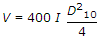is used for determining the velocity of ground water flow in metres per day. It is known as

A.
 Meinzer's formulaB.
 Slichter's formulaC.
 Darcy's formulaD.
 Hazen's formula.9.

In the derivation of Thiem's formula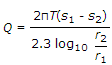, the following assumption is not applicable

A.
 The aquifer is homogeneous and isotropicB.
 Flow lines are radial and horizontalC.
 The slope of the water surface is too smallD.
 The well has been sunk up to the surface of the unconfined aquiferE.
 None of these.10.

Consumptive use of a crop during growth, is the amount of

A.
 interceptionB.
 transpirationC.
 evaporationD.
 all the above.11.

The best instrument for measuring the velocity of a stream flow is

A.
 pitot tubeB.
 Price's current meterC.
 surface floatD.
 sub-surface float.12.

The average mean velocity of a stream having depth h, may be obtained by taking the average of the readings of a current meter at a depth of

A.
 0.1 h and 0.9 hB.
 0.2 h and 0.8 hC.
 0.3 h and 0.7 hD.
 0.4 h and 0.6 h.13.

The best unit period of a unit hydrograph, is equal to basin lag divided by

A.
 2B.
 3C.
 4D.
 514.

While determining the yield of a gravity well by pumping, the depth of water table in two tests wells at distances r1 and r2 from the centre of the main well were found to be s1 and s2 respectively. Assuming the coefficient of transmissibility of the soil as T, the discharge Q may be given by

A.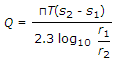B.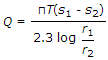C.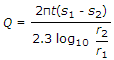D.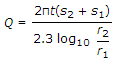15.

For high flood estimates the average value of the constant C in Dicken's formula Q = CA3/4, is

A.
 6.5B.
 8.5C.
 9.5D.
 10.5E.
 11.516.

Pick up the correct statement from the following :

A.
 Higher the gauge, more deficient will be the rain catchB.
 Heavier the rain, lesser will be the rain catchC.
 The trees serving as wind brakes in the vicinity of the gauge, should not subtend angles greater than 45°D.
 Coniferrous forest is idealy suited for installing the gaugeE.
 All the above.17.

The respective storm totals at three surrounding stations A, B and C are 110, 90 and 70 mm. If the normal annual precipitation amounts at stations X, A, B and C are respectively 1000, 1100, 1200 and 1250 mm, the estimated storm precipitation at X is

A.
 75 mmB.
 77 mmC.
 79 mmD.
 81 mm.18.

If the axial length of a drainage basin is 35 km and its form factor is 0.2, the total area of the basin is

A.
 205 sq. km.B.
 215 sq. km.C.
 225 sq. km.D.
 235 sq. km.E.
 245 sq. km.19.

Pick up the correct statement from the following :

A.
 The unit hydrograph of a specified unit duration obtained from the past data can be used to obtain the hydrograph of future storms of like durationB.
 To obtain the ordinates of storm hydrograph, the ordinates of unit hydrograph are multiplied by the multiplying factorC.
 The multiplying factor for storm hydrograph may be obtained by dividing the run off in mm by 25 mmD.
 A tolerance of about 25% of specified duration of unit hydrograph may be accepted without any serious errorE.
 All the above.20.

If the loss due to friction in pipes is 4 m, the total losses in strainer and bends may be taken as

A.
 0.10 mB.
 0.20 mC.
 0.5 mD.
 1.0 m.